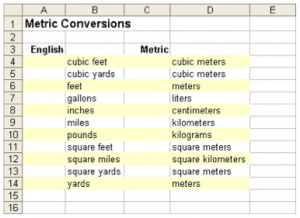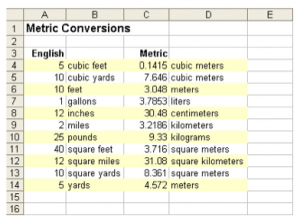# Metric

Although the metric system (also called SI) is used throughout the world, the U.S. still widely depends

on the English system of measurements.

a) Create a new workbook.

b) Enter the data and apply formatting as shown below:c) Save the workbook naming it Metric Conversions.

d) Use the information below to add the appropriate formulas that use cell references into cells C4 through C14:

• cubic meters = 0.0283*cubic feet
• cubic meters = 0.7646*cubic yards
• meters = 0.3048*feet
• meters = 0.9144*yards
• liters = 3.7853*gallons
• centimeters = 2.54*inches
• kilometers = 1.6093*miles
• kilograms = 0.3732*pounds
• square meters = 0.0929*square feet
• square kilometers = 2.59*square miles
• square meters = 0.8361*square yards

e) Check your spreadsheet by entering 5 in cell A4. Cell C4 should display 0.1415 because it automatically calculates the metric equivalent of 5 cubic feet.

f) Enter the following measurements into cells A4 through A14. Your worksheet should look similar to:g) Create a header that right aligns your name and a footer that center aligns the current date.

h) Save the modified Metric Conversions.

i) I will check the formulas when I grade them in class.